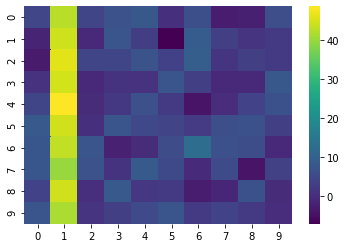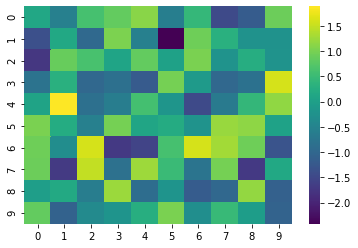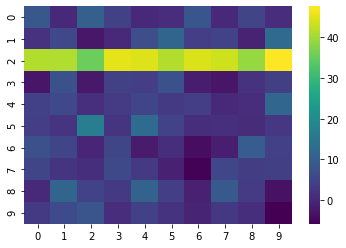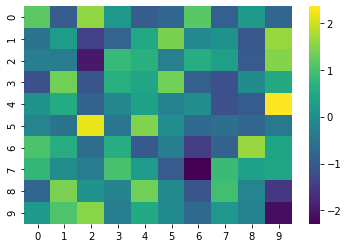In the first chart of the first example, you can see that while one column appears as yellow, the rest of the heatmap appears as green. This column absorbs all the color variations. To avoid this, you can normalize the data frame. You can normalize on columns or on rows. Several formula can be used, read this page to find the one you need.

## Column normalization

You can compare the charts below in order to see the difference between the initial data frame and the normalized version of it.

``````# libraries
import seaborn as sns
import matplotlib.pyplot as plt
import pandas as pd
import numpy as np

# Create a dataframe where the average value of the second column is higher than others:
df = pd.DataFrame(np.random.randn(10,10) * 4 + 3)
df=df+40

# If we do a heatmap, we just observe that one column has higher values than others:
sns.heatmap(df, cmap='viridis')
plt.show()

# Now if we normalize it by column:
df_norm_col=(df-df.mean())/df.std()
sns.heatmap(df_norm_col, cmap='viridis')
plt.show()``````## Row normalization

The same principle works for row normalization.

``````# libraries
import seaborn as sns
import matplotlib.pyplot as plt
import pandas as pd
import numpy as np

# Create a dataframe where the average value of the second row is higher
df = pd.DataFrame(np.random.randn(10,10) * 4 + 3)
df.iloc=df.iloc+40

# If we do a heatmap, we just observe that one row has higher values than others:
sns.heatmap(df, cmap='viridis')
plt.show()

# Normalize it by row:
df_norm_row = df.apply(lambda x: (x-x.mean())/x.std(), axis = 1)

# And see the result
sns.heatmap(df_norm_row, cmap='viridis')
plt.show()``````## Contact & Edit

👋 This document is a work by Yan Holtz. You can contribute on github, send me a feedback on twitter or subscribe to the newsletter to know when new examples are published! 🔥